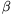Mathematical and Physical Journal
for High Schools
Issued by the MATFUND Foundation
 Already signed up? New to KöMaL?

# KöMaL Problems in Mathematics, May 2007

Show/hide problems of signs:## Problems with sign 'C'

Deadline expired on June 15, 2007.

C. 900. 75% of a certain three-digit number of different digits consists of the same digits as the original number but with no digit in the same place. Which number is it?

(5 pont)

solution (in Hungarian), statistics

C. 901. The area of a rectangle ABCD is. Let P denote the point on side AB, closer to A, that divides AB in a 1:4 ratio. Given that the line segment PD is perpendicular to diagonal AC, calculate the perimeter of the rectangle.

(5 pont)

solution (in Hungarian), statistics

C. 902. A circle K is touched on the inside by a circle k of half the radius. Construct a chord in K, perpendicular to the line connecting the centres of the circles that is divided into three equal parts by its intersections with k.

(5 pont)

solution (in Hungarian), statistics

C. 903. Find the largest value of the expression(5 pont)

solution (in Hungarian), statistics

C. 904. Find the acute anglesandthat satisfy the following simultaneous equations:(5 pont)

solution (in Hungarian), statistics## Problems with sign 'B'

Deadline expired on June 15, 2007.

B. 4002. Prove that if n>1 is an integer then there exists a sequence of integers a1=n<a2<a3<..., such that a12+...+ak2 is divisible by the number a1+...+ak for all values of k.

(3 pont)

solution (in Hungarian), statistics

B. 4003.are given points in the plane, no three of which are collinear. Show that for all 1i2007 there is an even number of triangles PjPkPl that contain Pi in the interior.

(British competition problem)

(5 pont)

solution (in Hungarian), statistics

B. 4004. A' is the reflection of the vertex A of an equilateral triangle ABC about the opposite side. A line passing through A' intersects the lines AB and AC at the points C' and B', respectively. What is the locus of the intersection of lines BB' and CC'?

(4 pont)

solution (in Hungarian), statistics

B. 4005. For every positive integer n, let an denote the number of ways n is obtained as a sum of terms that are all 1, 3 or 4. The order of the terms also matters. Prove that a2006a2007a2008 is a perfect cube.

(4 pont)

solution (in Hungarian), statistics

B. 4006. a, b, c are the sides of a triangle, such that a+b=2c. Prove that the centres of the inscribed and circumscribed circles are concyclic with the midpoints of the sides a and b.

(4 pont)

solution (in Hungarian), statistics

B. 4007. Let A, B, C, D denote four distinct points in the space. The line segment PQ is moved'' from AB to DC so that in each time instant P divides the line segment AD in the same ratio as Q divides BC. Then the line segment PQ sweeps out a surface. Prove that the same surface will be obtained if a line segment RS is moved in the same way from AD to BC.

(4 pont)

solution (in Hungarian), statistics

B. 4008. Prove that(3 pont)

solution (in Hungarian), statistics

B. 4009. The angle bisectors drawn from the vertices A and B of a triangle ABC intersect the opposite sides at A1 and B1, respectively. P is the intersection of the ray A1B1 with the circumscribed circle of the triangle. Prove that(5 pont)

solution (in Hungarian), statistics

B. 4010. p(x) and q(x) are polynomials of real coefficients that have no real roots in common, and p(q(x))=q(p(x)) for all x. Prove that the polynomials p(p(x)) and q(q(x)) have no common real roots either.

(4 pont)

solution (in Hungarian), statistics

B. 4011. Assoc City has 2007 inhabitants. They like joining clubs. The following two resolutions are passed to regulate club life: (1) every club must have exactly 101 members, and (2) no two clubs may have exactly the same set of members. In the senate of the city, each club is represented by a member, and it is against the law for two clubs to have the same representative. The committee of law are planning to impose a limitation on the number of clubs, so that however they are formed according to the rules above, it should always be possible to delegate representatives. What is the maximum number of clubs that may be allowed by the committee?

(5 pont)

solution (in Hungarian), statistics## Problems with sign 'A'

Deadline expired on June 15, 2007.

A. 428. Given a convex lattice polygon such that both x- and y-coordinates of each vertex lie in the interval [0,n]. Show that the number of vertices is less than 100n2/3.

(5 pont)

solution, statistics

A. 429. Find all pairs f(x), g(x) of polynomials with integer coefficients satisfying

f(g(x))=x2007+2x+1.

(Proposed by Katalin Gyarmati)

(5 pont)

solution, statistics

A. 430. Let n2 and letbe arbitrary complex numbers with absolute values at most 1 and let

f(x)=(x-u1)(x-u2)...(x-un).

Prove that polynomial f'(x) has a root with a non-negative real part.

(5 pont)

solution, statistics